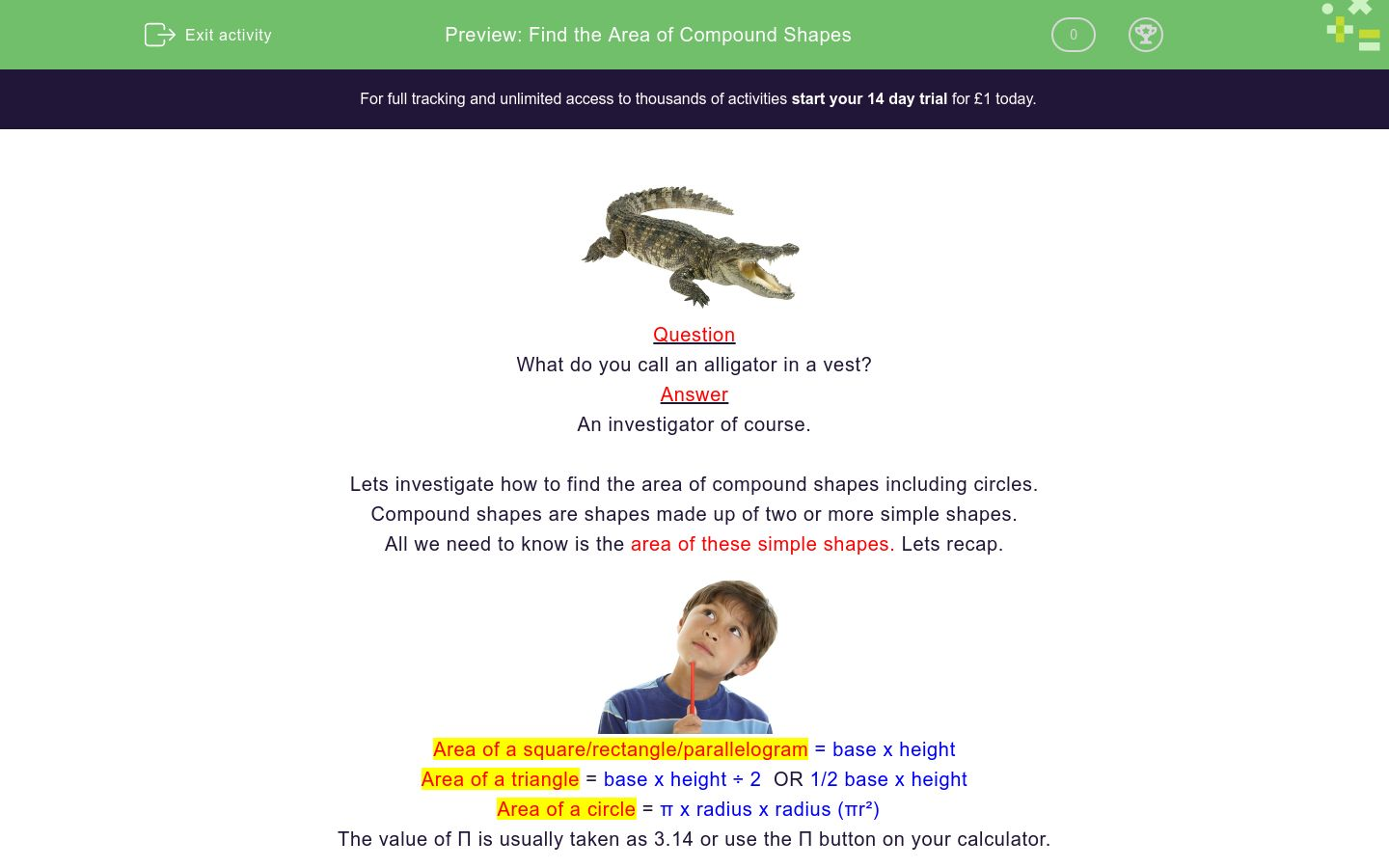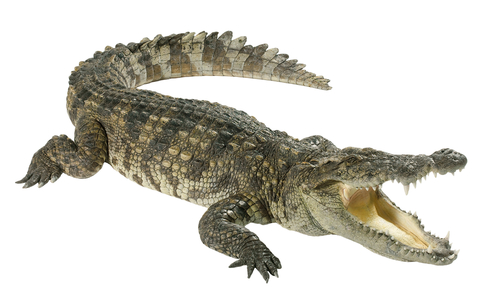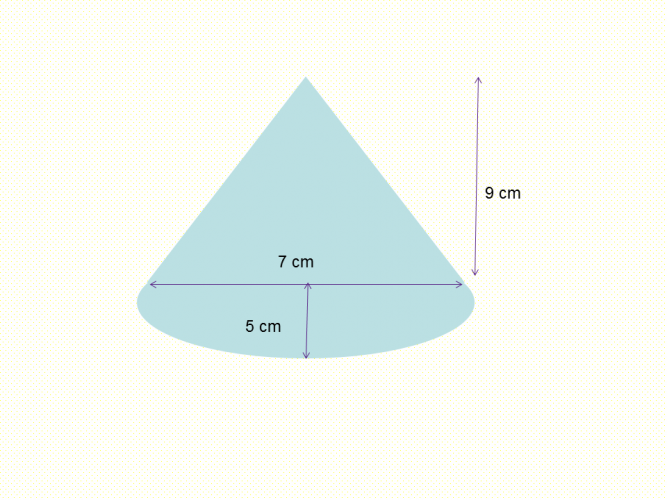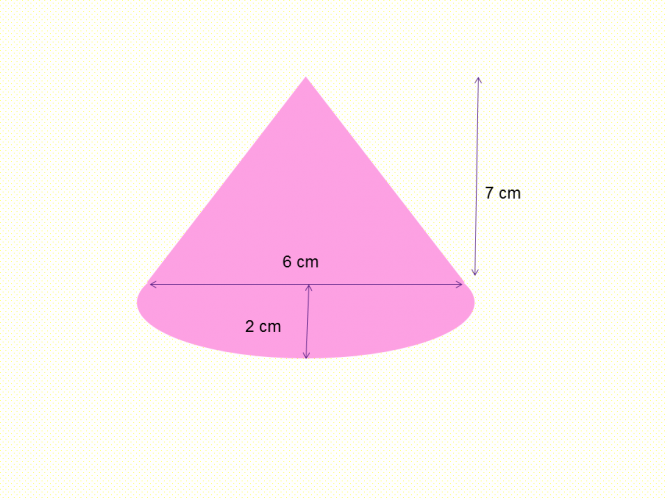# Find the Area of Compound Shapes

In this worksheet, students will find the area of compound shapes, including circles.Key stage:  KS 4

GCSE Subjects:   Maths

GCSE Boards:   AQA, Eduqas, Pearson Edexcel, OCR

Curriculum topic:   Geometry and Measures, Mensuration

Curriculum subtopic:   Mensuration and Calculation, Volume and Surface Area Calculations

Difficulty level:### QUESTION 1 of 10Question

What do you call an alligator in a vest?

An investigator of course.

Lets investigate how to find the area of compound shapes including circles.

Compound shapes are shapes made up of two or more simple shapes.

All we need to know is the area of these simple shapes. Lets recap.Area of a square/rectangle/parallelogram = base x height

Area of a triangle = base x height ÷ 2  OR 1/2 base x height

The value of Π is usually taken as 3.14 or use the Π button on your calculator.

Example 1Here you can separate this shape into a rectangle measuring 9 cm x 8 cm = 72 cm² and a semi circle.

To find the semi circle find the area of the full circle π x 3 x 3 =  28.27 now divide by 2 =  14.14 cm²

Now just add 72 cm² to 14.14 cm² = 86.14 cm²

Example 2Area of the triangle = 7 x 9 = 63 cm² ÷ 2 = 31.5 cm²

Area of the semi circle = π x 5 x 5 = 78.54 cm² ÷ 2 = 39.27 cm²

Total area = 31.5 cm² + 39.27 cm ² = 70.77 cm²What is the area of this shape?

The the answer has been rounded to 2 decimal places (2 d.p)

25.14 cm²

171.27 cm²

75.41 cm²

146.14 cm²Calculate the area of this shape.352.47 cm² 261.62 cm² 129.81 cm² The area of this shape isCalculate the area of this shape.

 352.47 cm² 261.62 cm² 129.81 cm² The area of this shape is100.45 cm² 90 cm² 245.96 cm² 45 cm² 190.48 cm² 200.96 cm² 100.48 cm² The area of the triangle is The area of the semi circle is Total area isFind the area of the shaded green part of the square.58 cm² 108.24 cm² 66.57 cm² 102.65 cm² The area of this shapes isFind the area of this shape.Find the area of the pink shaded triangle.

110.42 cm²

78.54 cm²

122.54 cm²

34.54 cm²Find the area of the orange shape.

110.42 cm²

78.54 cm²

122.54 cm²

34.54 cm²

• Question 1What is the area of this shape?

The the answer has been rounded to 2 decimal places (2 d.p)

146.14 cm²
EDDIE SAYS
The thing here is to remember tho halve the area of the circle to get the semi circle. This is the bit lots of people forget. The area of the rectangle is 11 x 12 = 121 cm ². The area of the semi circle is Π x 4 x 4 = 50.27 cm² 50.27 cm² ÷ 2 = 25.14 cm² Total area = 121 cm² + 25.14 cm² = 146.14 cm²
• Question 2Calculate the area of this shape.

27.28
EDDIE SAYS
Area of the triangle = 6 x 7 ÷ 2 = 21 cm² Area of the semi circle = Π x 2 x 2 = 12.57 ÷2 = 6.28 cm² Total area = 21 + 6.28 = 27.28 cm² correct to 2 decimal places.
• Question 3352.47 cm² 261.62 cm² 129.81 cm² The area of this shape is
EDDIE SAYS
This was a little sneaky wasn't it. There was no radius given. We could find the diameter of the circle 14 - 5 = 9 cm and then halve it to get the radius of 4.5 cm. Did you spot it? Area of the rectangle = 7 x 14 = 98 cm² Area of the semi circle = Π x 4.5 x 4.5 = 163.62 ÷ 2 = 31.81 cm² Total area = 98 + 31.81 = 129.81 cm² correct to 2 decimal places.
• Question 4Calculate the area of this shape.

EDDIE SAYS
Are you getting used to applying two formulas and adding them up now? Area of the rectangle = 25 x 32 = 800 cm² Area of the semi circle = Π x 12 x 12 = 452.39 ÷ 2 = 226.19 cm² Total area = 800 + 226.19 = 1026.19 cm² correct to 2 decimal places.
• Question 5100.45 cm² 90 cm² 245.96 cm² 45 cm² 190.48 cm² 200.96 cm² 100.48 cm² The area of the triangle is The area of the semi circle is Total area is
EDDIE SAYS
Area of the triangle = 10 x 9 ÷ 2 = 45 cm² Area of the semi circle = Π x 8 x 8 = 200.96 ÷ 2 = 100.48 cm² Total area = 45 + 45 + 100.48 = 190.48 cm² correct to 2 decimal places. Did you remember that there were two triangles, but you only had to calculate the area of one. As no other information given on the second triangle we have to assume they are the same size.
• Question 6Find the area of the shaded green part of the square.

42.06
EDDIE SAYS
Whoa..how do i find the area of those 4 triangle things? Area of the square 14 x 14 = 196 cm² Area of the circle = Π x 7 x 7 = 153.94 cm² Because you only want the area of the green shaded part you subtract the area of the circle from the area of the square. Total area = 196 - 153.94 = 42.06 cm² correct to 2 decimal places.
• Question 758 cm² 108.24 cm² 66.57 cm² 102.65 cm² The area of this shapes is
EDDIE SAYS
This is straight forward if you know your formulas and spotted that the circle gave you a diameter and not a radius. Area of parallelogram 9 x 6 = 54 cm² Area of circle = π x 2 x 2 = 12.57 cm², Total area = 54 + 12.57 = 66.57 cm²
• Question 8Find the area of this shape.

104.55
EDDIE SAYS
Strange looking shape isn't it. Area of the triangle = 8 x 12 ÷ 2 = 48 cm² Area of the semi circle = Π x 6 x 6 = 113.10 ÷ 2 = 56.55 cm² Total area = 48 + 56.55 = 104.55 cm² correct to 2 decimal places.
• Question 9Find the area of the pink shaded triangle.

34.54 cm²
EDDIE SAYS
Quite often you will have to subtract when working out areas. Make sure you can spot when. Area of the triangle = 8 x 11 ÷ 2 = 44 cm² Area of the circle = Π x 5 x 5 = 78.54 cm² Total area = 78.54 - 44 = 34.54 cm² correct to 2 decimal places.
• Question 10Find the area of the orange shape.

EDDIE SAYS
And finally.... Area of rectangle = 10 x 14 = 140 cm² The white part is a semi circle with a radius of 4 cm. Area of the semi circle = Π x 4 x 4 = 50.27 ÷ 2 = 25.14cm² Total area = 140 - 25.14 = 114.86cm² correct to 2 decimal places.
---- OR ----

Sign up for a £1 trial so you can track and measure your child's progress on this activity.

### What is EdPlace?

We're your National Curriculum aligned online education content provider helping each child succeed in English, maths and science from year 1 to GCSE. With an EdPlace account you’ll be able to track and measure progress, helping each child achieve their best. We build confidence and attainment by personalising each child’s learning at a level that suits them.

Get started